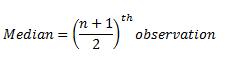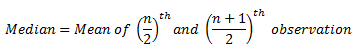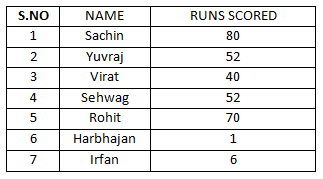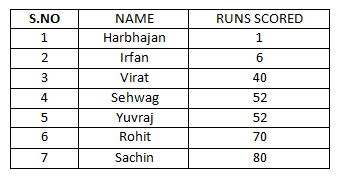# Median Of Data And Statistics

Median of Data

Statistics deals with the study of analysis, presentation, interpretation, organisation and collection of data. A basic procedure for statistics includes the test of relationship between a synthetic data and statistical data drawn from a idealized model. There are several methods of representing a set of data through polygons, bar graphs, pie-charts, frequency, graphs, pictorial representation of histograms, tables etc.

After the data is organized and represented in suitable form, the data is used for analysis. Measures of Central Tendency is used to utilize systematized data and to obtain symbolic results from obtained data. With the help of measures of central tendency, arbitrary of vast organization of data can be made. The entire data will be briefed into one particular value that represents the aspects of the complete organized data.

They are several specifications that are used as measures of central tendency namely mode, median and mean. Let us discuss in brief about importance of median of data.

##### Median of Data

To calculate the median of a set of data, the observations are arranged in ascending or descending order and then the middle or central value of the set of observations gives us the median of data.

Based upon number of observations, two cases can arise i.e. either the total number of observations will be odd or they would be even. Median in both the cases is determined using different formula.

When the set of data has odd number of observations then;When the set of data has even number of observations then the median is;Let us look into an example to understand the concept of median properly;

Example: The below table illustrates the scores obtained by each players in a match. Calculate mean, median and mode of the given data?To determine the median of data let us arrange the given data in ascending orderSince the number of observations in the data is odd the median is ((n+1)/2)th observation.

⇒Median = ((7+1)/2)th observation

⇒Median = 4th observation = 52